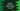# Python program to convert an integer number to octal## How to convert an integer number to octal string in python :

Python comes with one built-in method_ oct()_ for converting an integer number to octal easily. You need to pass one integer number to this method. It will convert the integer to octal string and returns the value. One thing you should note that the returned octal string is prefixed with 0o. If the parameter value is not a python int, it should define_ index()_ method that returns an integer.

The syntax of the oct() method is as below :

``oct(x)``

where, x _is an _integer number in binary, decimal or hexadecimal _format. As explained above, the return value is an octal representation of the integer number _x.

### Example :

Let’s try oct() with a binary, decimal and _hexadecimal _number.

``````print("octal representation of 10 is ", oct(10))
print("octal representation of 0xC is ", oct(0xC))
print("octal representation of 0b1010 is ", oct(0b1010))``````

It will print out the below output :

``````octal representation of 10 is  0o12
octal representation of 0xC is  0o14
octal representation of 0b1010 is  0o12``````

### Explanation :

1. The first number is decimal 10. Octal representation of 10 is 12. Note that the output is prefixed with 0o.
2. The second number is the hexadecimal representation of 12. Octal representation of 12 is 14. So, the result is 0o14.
3. The last number is a binary number, which is the binary representation of 10. This is the reason the output of the first and the third line is the same.

We have tried oct() with decimal, hexadecimal and binary number in the above example. Now, let’s try it with a custom object.

### Find oct() for a custom object :

As mentioned before, for a custom object, we need to implement_ index()_ method, that will return an integer if we want to find the octal value for that object. Let’s do that :

``````class Student:
rank = 73

def __index__(self):
return self.rank

def __int__(self):
return self.rank

student = Student()
print("oct value of \'student\' is", oct(student))``````

#### Output :

``oct value of 'student' is 0o111``

The above program is also available on Github.

### Conclusion :

octal conversion is easy to implement in python using oct() method. Go through the examples above and try to run each one on your machine. If you have any queries, drop a comment below.